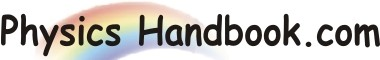HOME TOPICS DEFINITIONS TABLES LAWS INVENTIONS EXPERIMENTS QUIZ VIDEOS
 A B C D E F G H I J K L M N O P Q R S T U V W X Y Z
Chain Reaction
The flow of charged particles in a definite direction is called electric current. Those substances which permit the flow of charges through them are called conductors e.g. metals. However, those substances which do not permit the flow through a conductor if p.d. is maintained across its ends.
(I) Current Carrier in Solid Conductors : In solid conductors (e.g. metals), there are a large number of free electrons. when electric field (i.e. pd) is applied to the conductor, the free electrons start drifting in a particular direction to constitute that current will only flow through a conductor if p.d. is maintained across its ends.
(II) Current Carrier in Liquids : Some liquids are conductors of electricity. A Conducting liquid is called an electrolyte (e.g. solution of CuSO4). The electrolytic solution provides positive ions (e.g. Cu++) and negative ions (e.g. SO4--).When external electric field (i.e. p.d.) is applied, the positive ions move in one direction and negative ions in the opposite direction to constitute electric current. Hence in conducting liquids, ions (positive and negative) are the current carriers.
(II) Current Carrier in Gases : Under ordinary conditions, gases are insulators. However, when a gas under low pressure is subjected to high electric field (i.e. high p.d.), Ionisation of in gases molecules take place, i.e. electrons and positive ions are formed. Hence, current carriers in gases are free electrons and positive ions.